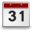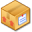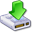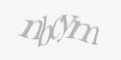0
0
0
s2sdefault

### Article Index

I have been having connectivity issues of a strange variety at home. I have called the ISP and had many discussions, and frankly, some argumants on my connectivity to the internet. The ISP, like all others, claims that it isn't their fault, and must be something with my wireless router, or other problem with my network and my setup. Myself, knowing exactly how this is all setup, I know this to be not the case. Or at least I am very reasonably sure...

Since there is debate over the source of the failure, I have written a little script that does a couple of pings, and logs the results to a file. In the end, there are 2 pings, one sent to the default gateway, and one sent to google.ca.This will help narrow down any possible failures... If I am able to ping google.ca, the internet connectivity is fine. If I can ping my default gateway, but not google, then the internet connection itself is the problem. And if I cannot ping the gateway, then there's the problem there. Again, none of this is very useful without any date/time data, so we need that. And since I am using a ping, I also want to get the return time to serve as a (minor) basis for connectivity speed.

There are probably many other ways to accomplish the same goals I am after here, but since I have the ability to do so, I wrote up my own script for this. I have tested this script here in my lab for a few days, and I think it works as expected. Though only a little more "real-world" testing will help dis/prove this. Let's take a look at the script:

`#! /bin/bash`

`PING_TARGET="google.ca"`

`LOG_DIR=/var/log/conn`

`LOG_FILE=\$LOG_DIR"/conn.log"`

`PING_COMMAND="ping -W 1 -c 1"`

`if [ "`id -u`" != "0" ]`

`then`

`    echo "You need root privileges!"`

`    exit`

`fi`

`if [ ! -e \$LOG_DIR ]`

`then`

`    mkdir \$LOG_DIR`

`fi`

`if [ ! -e \$LOG_FILE ]`

`then`

`    touch \$LOG_FILE`

`    chmod 757 \$LOG_FILE`

`    echo "Date/Time, Uptime (in secs), CPU Load, Next Hop IP, Ping Time (in ms), Target IP, Ping Time (in ms)," >> \$LOG_FILE`

`fi`

`COUNTER=1`

`while [ \$COUNTER -le 6 ]`

`do`

`    FINAL_LOG=\$(date),`

`    UPTIME=\$(cat /proc/uptime | awk '{ print \$1 }') # uptime in secs`

`    FINAL_LOG+=\$UPTIME,`

`    CPU=\$(cat /proc/loadavg | awk '{print \$1}')`

`    FINAL_LOG+=\$CPU,`

`    DEFAULT_ROUTE=\$(/sbin/route | grep default | awk '{ print \$2 }')`

`    wait`

`    if [ -z "\$DEFAULT_ROUTE" ]`

`    then`

`        FINAL_LOG+="No Default Route, ,";`

`    else`

`        PING_DR="\$(\$PING_COMMAND \$DEFAULT_ROUTE 2>&1)"`

`        DR_IP=\$(awk -F " " '{print \$3;}' <<< \$PING_DR) `

`        FINAL_LOG+=\$DR_IP,`

`        DRPINGTIME=\$(awk -F " " '{print \$14;}' <<< \$PING_DR)`

`        DRPINGTIME=\${DRPINGTIME:5}`

`        FINAL_LOG+=\$DRPINGTIME,`

`    fi`

`    PING_OUTPUT="\$(\$PING_COMMAND \$PING_TARGET 2>&1)"`

`    if [[ \$PING_OUTPUT == *"unknown host"* ]]`

`    then`

`        FINAL_LOG+="DNS ERROR, ,";`

`    elif [[ \$PING_OUTPUT == *"0 received"* ]]`

`    then`

`        FINAL_LOG+="Ping Timeout, ,";`

`    else`

`        PING_IP=\$(awk -F " " '{print \$3;}' <<< \$PING_OUTPUT) `

`        FINAL_LOG+=\$PING_IP,`

`        PINGTIME=\$(awk -F " " '{print \$15;}' <<< \$PING_OUTPUT)`

`        PINGTIME=\${PINGTIME:5}`

`        FINAL_LOG+=\$PINGTIME,`

`    fi`

`    echo \$FINAL_LOG >> \$LOG_FILE`

`    COUNTER=\$(( COUNTER+1 ))`

`    sleep 10`

`done`

Let's break the script down...

DEFINE VARIABLES

`PING_TARGET="google.ca"`

`LOG_DIR=/var/log/conn`

`LOG_FILE=\$LOG_DIR"/conn.log"`

Here, I am just setting a few variables up near the top of the script. It makes changing them a little easier.

`PING_COMMAND="ping -W 1 -c 1"`

This is the basic command that I will be using.

-W 1 - set a timeout on the ping for 1000 miliseconds (1 second)

-c 1 - send only 1 ping

LOG DIRECTORY AND FILE

`if [ "`id -u`" != "0" ]`

`then`

`    echo "You need root privileges!"`

`    exit`

`fi`

`if [ ! -e \$LOG_DIR ]`

`then`

`    mkdir \$LOG_DIR`

`fi`

`if [ ! -e \$LOG_FILE ]`

`then`

` touch \$LOG_FILE`

`    chmod 757 \$LOG_FILE`

`    echo "Date/Time, Uptime (in secs), CPU Load, Next Hop IP, Ping Time (in ms), Target IP, Ping Time (in ms)," >> \$LOG_FILE`

`fi`

First, check to make sure the script is being run with root privileges. Then, check for the log directory then the log file; if either is missing, create.

LOOP

`COUNTER=1`

`while [ \$COUNTER -le 6 ]`

`do`

` ...`

` COUNTER=\$(( COUNTER+1 ))`

` sleep 10`

`done`

I want my script to send a series of pings every 10 seconds. Since cron jobs can only run every minute at max, I need to set up a loop to run the commands for a total of 6 times, every 10 seconds. That is what this loop consists of.

COMMANDS (all within the loop)

`FINAL_LOG=\$(date),`

Log the date/time stamp.

`UPTIME=\$(cat /proc/uptime | awk '{ print \$1 }')`

`FINAL_LOG+=\$UPTIME,`

Log the ping tester's uptime.

`CPU=\$(cat /proc/loadavg | awk '{print \$1}')`

`FINAL_LOG+=\$CPU,`

Log the device's current CPU usage. One of the possibilities here is that the issues *MAY* be (or partially) due to CPU load on the device. This logs the avg CPU usage for the past 60 seconds.

`DEFAULT_ROUTE=\$(/sbin/route | grep default | awk '{ print \$2 }')`

Log the default route IP.

`wait`

Wait for all the above commands to complete

`if [ -z "\$DEFAULT_ROUTE" ]`

Check if the default route exists.

`then`

`    FINAL_LOG+="No Default Route, ,";`

If not, log that info.

`else`

`    PING_DR="\$(\$PING_COMMAND \$DEFAULT_ROUTE 2>&1)"`

Ping the default gateway.

`    DR_IP=\$(awk -F " " '{print \$3;}' <<< \$PING_DR) `

`    FINAL_LOG+=\$DR_IP,`

Extract the IP address from the ping response and log.

`    DRPINGTIME=\$(awk -F " " '{print \$14;}' <<< \$PING_DR)`

`    DRPINGTIME=\${DRPINGTIME:5}`

`    FINAL_LOG+=\$DRPINGTIME,`

Extract the ping time to the default gateway.

`fi`

`PING_OUTPUT="\$(\$PING_COMMAND \$PING_TARGET 2>&1)"`

Ping the PING_TARGET

`if [[ \$PING_OUTPUT == *"unknown host"* ]]`

`then`

`    FINAL_LOG+="DNS ERROR, ,";`

If the ping output shows "unknown host", this would be due to a DNS failure. Log this info if this is the case

`elif [[ \$PING_OUTPUT == *"0 received"* ]]`

`then`

`    FINAL_LOG+="Ping Timeout, ,";`

If the ping timed out, log that info

`else`

`    PING_IP=\$(awk -F " " '{print \$3;}' <<< \$PING_OUTPUT) `

`    FINAL_LOG+=\$PING_IP,`

Log the actual IP Address we are pinging (since we are pinging a DNS name, the IP is likely to change).

`    PINGTIME=\$(awk -F " " '{print \$15;}' <<< \$PING_OUTPUT)`

`    PINGTIME=\${PINGTIME:5}`

`    FINAL_LOG+=\$PINGTIME,`

Again, extract and log the ping response time.

`fi`

`echo \$FINAL_LOG >> \$LOG_FILE`

Log the data to the log file.

I am sure that someone, somewhere will look at this script and cringe, or make faces... Likely because there already exists something that will do something somewhat similar.But I needed something very lightweight, requiring little to no install and setup, useable on just about any/all Linux machines, and using (as much as possible) built-in commands like ping. This is also serving as an exercise in Linux and shell scripting. So please, feel free to download it, look it over, poke and prod at it, and give me your thoughts below.Ping Connectivity 1 HOTMonday, 07 September 2015 15:571.17 KB137 Download